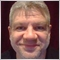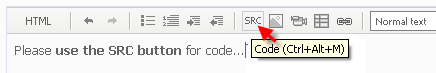# Indicator multi color - Refresh problem38

Hi all,

i've created an indicator with three buffers to manage color changing, but i've a problem about refresh of the last candle (candle in realtime)...don't work correctly in that candle (it's not enough a "Windowredraw" instruction).

Thanks for suggest.42595

Piratone:

Hi all,

i've created an indicator with three buffers to manage color changing, but i've a problem about refresh of the last candle (candle in realtime)...don't work correctly in that candle (it's not enough a "Windowredraw" instruction).

Thanks for suggest.38

int start()
{
int    nLimit,nCountedBars;
string Y,M,D,Giorno;

nCountedBars=IndicatorCounted();
if(nCountedBars>0) nCountedBars--;
nLimit=Bars-nCountedBars-1;
//----
for(i=nLimit; i>=0; i--)
{
Y=IntegerToString(TimeYear(Time[i]));
M=IntegerToString(TimeMonth(Time[i]));
D=IntegerToString(TimeDay(Time[i]));
Giorno=Y + "." + M + "." + D;
if(Time[i]==StrToTime(Giorno + " " + OffStart))
{
ExtOBVBuffer[i]=xxx;
ExtAppoggioBuffer[i]=xxx;
Vol=xxx;
Min=Vol;
Max=Vol;
Up=false;Dw=false;Nt=true;
continue;
}

if(i==Bars-1)
{
ExtOBVBuffer[i]=xxx;
ExtAppoggioBuffer[i]=xxx;
}
else
{
double dCurrentPrice=GetAppliedPrice(COHLMTW, i);
double dPreviousPrice=GetAppliedPrice(COHLMTW, i+1);
if(dCurrentPrice==dPreviousPrice)
{
Vol=Vol;
}
else
{
if(dCurrentPrice<dPreviousPrice)
{
if(Nt)Vol=ExtOBVBuffer[i+1];
if(Up)Vol=ExtOBVBufferUp[i+1];
if(Dw)Vol=ExtOBVBufferDw[i+1];
}
else
{
if(Nt)Vol=ExtOBVBuffer[i+1];
if(Up)Vol=ExtOBVBufferUp[i+1];
if(Dw)Vol=ExtOBVBufferDw[i+1];
}
}
}

if(Vol>=Min+DeltaPuntiInd){Up=true;Dw=false;Nt=false;Min=Vol;}
if(Vol<=Max-DeltaPuntiInd){Up=false;Dw=true;Nt=false;Max=Vol;}

if(Nt){ExtOBVBuffer[i]=Vol;ExtOBVBufferUp[i]=EMPTY_VALUE;ExtOBVBufferDw[i]=EMPTY_VALUE;}
if(Up){ExtOBVBuffer[i]=EMPTY_VALUE;ExtOBVBufferUp[i]=Vol;ExtOBVBufferDw[i]=EMPTY_VALUE;}
if(Dw){ExtOBVBuffer[i]=EMPTY_VALUE;ExtOBVBufferUp[i]=EMPTY_VALUE;ExtOBVBufferDw[i]=Vol;}

if(Vol<Min)Min=Vol;
if(Vol>Max)Max=Vol;

ExtAppoggioBuffer[i]=Vol;

WindowRedraw();
}
//----
return(0);
}Moderator
32147

Piratone:

Hi all,

i've created an indicator with three buffers to manage color changing, but i've a problem about refresh of the last candle (candle in realtime)...don't work correctly in that candle (it's not enough a "Windowredraw" instruction).

Thanks for suggest.

Your post is not very clear, but I have an idea of what is causing your problem.

When your code is checking conditions for Bar, conditions can change from tick to tick. This can result in more than 1 buffer receiving a value for that bar.

You can get over this by setting all buffers on Bar to EMPTY_VALUE before checking the conditions.

The same goes for Bar because it is possible that the last tick is missed when it was Bar and so it needs to be checked that conditions did not change on the last tick.25838

Piratone: i've a problem about refresh of the last candle (candle in realtime)...don't work correctly in that candle
```if(Nt)Vol=ExtOBVBuffer[i+1];
if(Up)Vol=ExtOBVBufferUp[i+1];
if(Dw)Vol=ExtOBVBufferDw[i+1];```
1.Play video Please edit your post. For large amounts of code, attach it.

2. In the initial fill Nt/Up/Dn contains the state of the previous candle and is used to compute the next. At the start of the loop you need to set those variables to the state they were at the previous candle. You're currently using the last calculated state of the current candle.38

Thanks Gum,

i've tried to insert this instructions:

ExtOBVBuffer[i]=EMPTY_VALUE;
ExtOBVBufferUp[i]=EMPTY_VALUE;
ExtOBVBufferDw[i]=EMPTY_VALUE;
ExtAppoggioBuffer[i]=EMPTY_VALUE;

before

if(Nt){ExtOBVBuffer[i]=Vol;ExtOBVBufferUp[i]=EMPTY_VALUE;ExtOBVBufferDw[i]=EMPTY_VALUE;}
if(Up){ExtOBVBuffer[i]=EMPTY_VALUE;ExtOBVBufferUp[i]=Vol;ExtOBVBufferDw[i]=EMPTY_VALUE;}
if(Dw){ExtOBVBuffer[i]=EMPTY_VALUE;ExtOBVBufferUp[i]=EMPTY_VALUE;ExtOBVBufferDw[i]=Vol;}

but not change.

if i don't use different color, so one buffer, all is ok.38

WHRoeder:
1.  Play video Please edit your post. For large amounts of code, attach it.

2. In the initial fill Nt/Up/Dn contains the state of the previous candle and is used to compute the next. At the start of the loop you need to set those variables to the state they were at the previous candle. You're currently using the last calculated state of the current candle.

Thanks WH, you've inspirated my mind :)

Next time, i'll use SRC button.